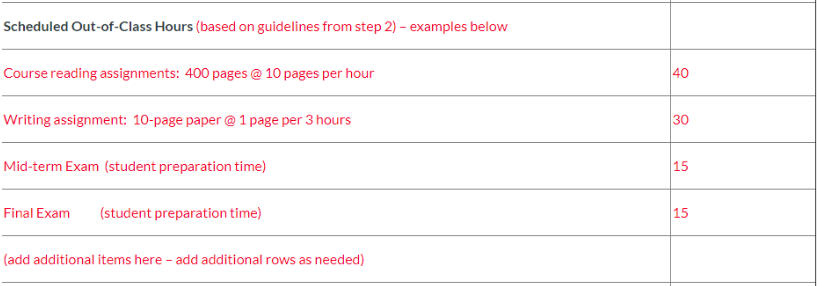Last Updated by:  Bennett Criss, May 2019

Part of setting up your course includes calculating your course's credit hours.  This documentation will show some recommendations for how to calculate the hours.

### Scheduled In-Class Hours:

To calculate your in-class hours, you need to take the number of hours per week that students are in class and multiply it by the number of course sessions in the semester (e.g. one 3 hour class per week * 13 class sessions = 39 in-class hours).  Please note that this is only "scheduled" in-class hours.  This does not include any additional class sessions or any cancelled sessions.

### Scheduled Out-of-Class Hours:

You can calculate your out-of-class hours by taking all out-of-class work (reading, quizzes, papers, etc.) and estimating the amount of preparation and completion time.  This is an estimate, since not every students complete work at the same pace.  The chart below from the course templates shows some examples of how to calculate the time for these assignments.### Total Student Work:

To calculate the total student work hours, just add up all the scheduled in-class hours and the scheduled out-of-class hours to get the total amount.